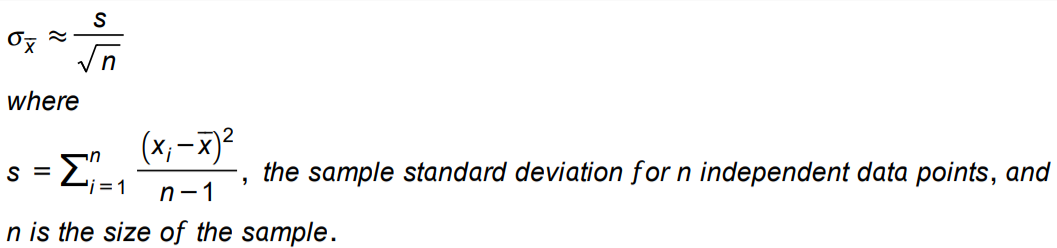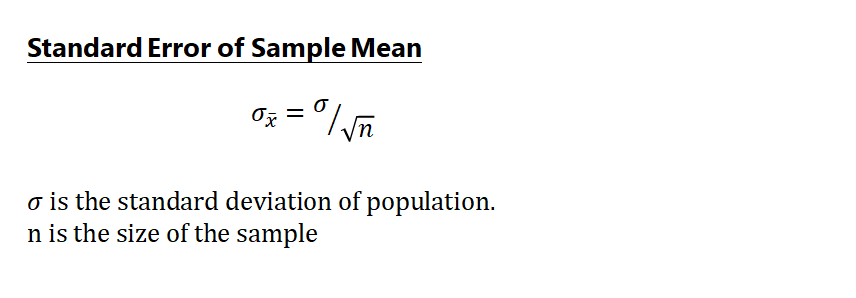21 Matching Annotations
1. Feb 2022
2. s3.us-west-2.amazonaws.com s3.us-west-2.amazonaws.com
1. 1

Note: 1. Eighty-four percent of autocracies from 1946 to 2010 had a ruling party (Cheibub, Gandhi, and Vreeland 2010), and 57 percent of these parties failed to outlive the founding leader (Meng 2019).

2. 2

Note: 2. I use the terms “authoritarian regime” and “dictatorship” synonymously. I also use the terms “dictator,” “authoritarian leader,” and “president” interchangeably.

#### URL

3. Jan 2022
4. s3.us-west-2.amazonaws.com s3.us-west-2.amazonaws.com
1. I claim that constitutional rules thatdesignate a formal successor play a critical role in promoting peaceful leadershiptransitions in dictatorships

to designate a formal successor

2. Figure 1. Autocratic leadership transitions, 1946 to 2014.

peaceful vs. unpeaceful power transitions:

From 1946 to 2014, only 44 percent of autocratic leadership transitions were peaceful and resulted in the continuation of the regime after the departure of the incumbent.

3. regimes that formally designate the vice president asthe successor are more likely to undergo peaceful transitions

leadership succession, authoritarian regime, constitutional rules, Africa

#### URL

5. Local file Local file
1. 1.1 Bernoulli distribution

$$Y \sim f_{B}(y ; \theta)= \begin{cases}\theta^{y}(1-\theta)^{1-y} & \forall y \in\{0,1\} \\ 0 & \text { otherwise }\end{cases}$$

$$E[Y]=\theta$$

$$var(Y)=\theta(1-\theta)$$

2. 1.6 conclusion

The key innovation in the likelihood framework is treating the observed data as fixed and asking what combination of probability model and parameter values are the most likely to have generated these specific data.

3. maximum likelihood: general

General Steps

• Step 1: Express the joint probability of the data.
• Step 2: Convert the joint probability into a likelihood.
• Step 3: Use the chosen stochastic and systematic components to specify a probability model and functional form.
• Step 4: Simplify the expression by first taking the log and then eliminating terms that do not depend on unknown parameters.
• Step 5: Find the extrema of this expression either analytically or by writing a program that uses numerical tools to identify maxima and minima.
4. Definition 1.1 (Sum of squared errors (SSE))

$$\mathrm{SSE}=\sum_{i=1}^{n}\left[y_{i}-\left(\beta_{0}+\beta_{1} x_{i}\right)\right]^{2}$$

\begin{aligned} &\hat{\beta}_{0}=\bar{y}-\hat{\beta}_{1} \bar{x} \\ &\hat{\beta}_{1}=\frac{\sum_{i=1}^{n}\left(y_{i}-\bar{y}\right)\left(x_{i}-\bar{x}\right)}{\sum_{i=1}^{n}\left(x_{i}-\bar{x}\right)^{2}} \end{aligned}

5. 1.4 Gaussian (normal) distribution

$$Y_i$$

is distributed iid normal with mean $$μ_i$$ and variance$$σ^2$$

$$Y \sim f_{\mathcal{N}}(y ; \boldsymbol{\theta})=\frac{1}{\sqrt{2 \pi \sigma^{2}}} \exp \left[-\frac{(y-\mu)^{2}}{2 \sigma^{2}}\right]$$

6. Rather than consider the data as random and the parameters asfixed, the principle of maximum likelihood treats the observed data as fixedand asks: “What parameter values are most likely to have generated thedata?”

maximum likelihood:

The MLEs are those that provide the density or mass function with the highest likelihood of generating the observed data.

7. 1.3 Bias and mean squared error

Let $$T(X)$$ be an estimator for $$\theta$$. The bias of $$T(X)$$, denoted $$\operatorname{bias}(\theta)$$, is $$\operatorname{bias}(\theta)=\mathrm{E}[T(X)]-\theta$$ The mean squared error, $$\operatorname{MSE}(\theta)$$, is given as \begin{aligned} \operatorname{MSE}(\theta) &=\mathrm{E}\left[(T(X)-\theta)^{2}\right] \ &=\operatorname{var}(T(X))+\operatorname{bias}(\theta)^{2} \end{aligned}

8. 1.2 Binomial distribution

\begin{aligned} X & \sim f_{b}(x ; n, p) \\ \operatorname{Pr}(X=k) &=\left\{\begin{array}{lll} \left(\begin{array}{l} n \\ k \end{array}\right) p^{k}(1-p)^{n-k} & \forall & k \in\{0, \ldots, n\} \\ 0 & \forall & k \notin\{0, \ldots, n\} \end{array}\right. \end{aligned}

where $$\left(\begin{array}{l}n \ k\end{array}\right)=\frac{n !}{k !(n-k) !}$$ and with $$\mathrm{E}[X]=n p$$ and $$\operatorname{var}(X)=n p(1-p)$$ The Bernoulli distribution is a binomial distribution with $$n=1$$.

9. The value of θ that the maximizes the likelihood function is called the maximumlikelihood estimate

Definition of MLE.

10. 4.2 Mixture distribution/mixturemodel

$$f\left(x ; w_{j}, \boldsymbol{\theta}_{j}\right)=\sum_{j=1}^{J} w_{j} g_{j}\left(x ; \boldsymbol{\theta}_{j}\right)$$

$$\mathcal{L}\left(w_{j}, \boldsymbol{\theta}_{j} \mid \mathbf{x}\right)=\prod_{i=1}^{n}\left[\sum_{j=1}^{J} w_{j} g_{j}\left(x_{i} ; \boldsymbol{\theta}_{j}\right)\right]$$

11. Definition 4.1 (Profile Likelihood)

\begin{aligned} \mathcal{L}_{p}\left(\boldsymbol{\theta}_{1}\right) & \equiv \max _{\boldsymbol{\theta}_{2}} \mathcal{L}\left(\boldsymbol{\theta}_{1}, \boldsymbol{\theta}_{2}\right) \\ & \equiv \mathcal{L}\left(\boldsymbol{\theta}_{1}, \hat{\boldsymbol{\theta}}_{2}\left(\boldsymbol{\theta}_{1}\right)\right) . \end{aligned}

12. 4.1 Uniform distribution

Uniform distribution: $$f(x)= \begin{cases}\frac{1}{b-a} & x \in[a, b] \ 0 & \text { otherwise }\end{cases}$$ $$E[x]={a+b\over2}$$ $$var(X)={(b-a)^2\over12}$$

#### Annotators

6. towardsdatascience.com towardsdatascience.com
1. Central Limit Theorem

the Central Limit Theorem tells us the sampling distribution of X̄ is closely approximated to a normal distribution.

2. the sample standard deviation S3. standard error4. Generally, bootstrap involves the following steps:
1. A sample from population with sample size n.
2. Draw a sample from the original sample data with replacement with size n, and replicate B times, each re-sampled sample is called a Bootstrap Sample, and there will totally B Bootstrap Samples.
3. Evaluate the statistic of θ for each Bootstrap Sample, and there will be totally B estimates of θ.
4. Construct a sampling distribution with these B Bootstrap statistics and use it to make further statistical inference, such as:
• Estimating the standard error of statistic for θ.
• Obtaining a Confidence Interval for θ.